Disclosure: This post may contain affiliate links, meaning when you click the links and make a purchase, we receive a commission.

# How to reference a cell from a different worksheet in Excel

Before understanding this topic, you have to understand cell reference in a formula. Say I have a formula like this ‘=B3*C3+D3’. In this formula there are three cell references, they are B3, C3, and D3. Every reference in a formula links with a cell in excel worksheet. In other words, every cell has a unique reference. B3 can link to only one cell in the worksheet.

You can use the cell reference of one worksheet with another worksheet. Say you have two worksheets in your excel workbook, they are ‘Menu Order’ and ‘Total Cost’. You want to use the value of cell F15 in ‘Menu Order’ worksheet in a cell of ‘Total Cost’ worksheet. To do this, you will use first the worksheet name in the formula, then add an exclamation sign (!) and at the end use the cell reference. Our formula will be like this = ‘Menu Order’!F15.

NOTE: If a worksheet name contains space, then you have to use single quotation marks(”) around the name, otherwise use the name direct in the formula.

## Using cell references with multiple worksheets in Excel

Using a real-time example, we shall make clear to you how to use cell references with multiple worksheets.

### Step 1:

In our example, we have two worksheets, they are ‘Menu Order’ and ‘Total Cost’. We shall use the cell reference of worksheet ‘Menu Order’ with a cell in our another worksheet ‘Total Cost’.
Select the cell and the worksheet name that you want to use in another worksheet. We have selected the cell F15 of ‘Menu Order’ worksheet.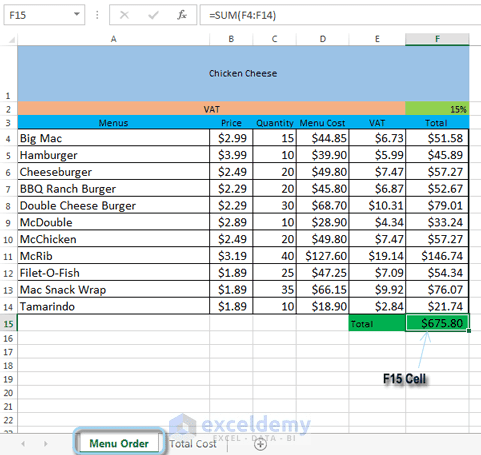Cell references with multiple worksheets. We have selected cell F15 in our ‘Menu Order’ worksheet.

### Step 2:

Now navigate to your desired worksheet. In our example, we have navigated to the ‘Total Cost’ worksheet.

### Step 3:

The selected worksheet will be visible.

### Step 4:

Select the cell where you will write your formula with another worksheet’s cell reference. We have selected cell B2.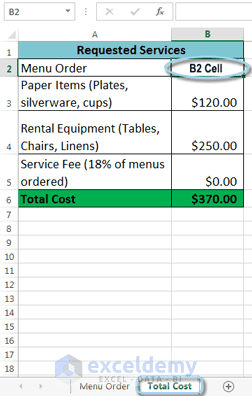Cell references with multiple worksheets. We have navigated to the ‘Total Cost’ worksheet.

### Step 5:

Double click on cell B2 in our example. Then type an equal sign (=), then the sheet name with an exclamation sign (!), then the cell reference. Our formula will be like this: =’Menu Order’!F15.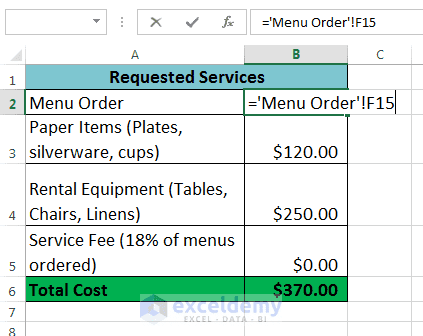Cell references with multiple worksheets. We have typed =’Menu Order’!F15 in cell B2.

### Step 6:

Press Enter. The value of the referenced cell will be visible. If you change the value of cell F15 on the ‘Menu Order’ worksheet, the B2 value will be updated automatically on the ‘Total Cost’ worksheet.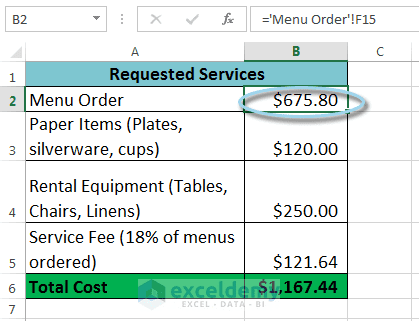Cell references with multiple worksheets. B2 is showing the value of F15 of ‘Menu Order’ worksheet.

Note 1: If you rename your worksheet name later, the formula will be updated automatically with the new name of the worksheet.

Note 2: If you type the worksheet name wrongly, then a #REF! error will be visible. Click the yellow error button to edit the worksheet name with ‘Edit in Formula Bar’ option.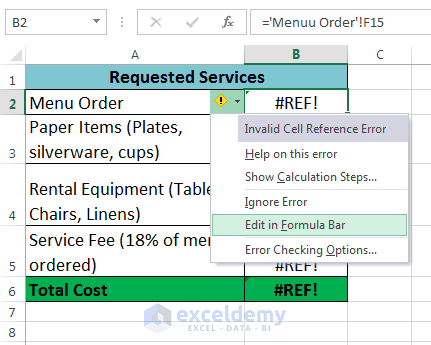Cell references with multiple worksheets. If you type worksheet name wrongly, then click on the yellow error button to edit it.